# Multiplicatively monotone norm is constant on associate classes

## Statement

Suppose$R$ is a commutative unital ring and$N$ is a norm on$R$ that is multiplicatively monotone: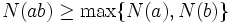$N(ab) \ge \max \{ N(a), N(b) \}$ whenever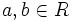$a,b \in R$ are such that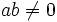$ab \ne 0$. Then, if$a,b \in R$ are associate elements, we have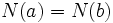$N(a) = N(b)$.

Note that the proof does not in fact use the fact that the norm is Euclidean.

## Proof

Given: A multiplicatively monotone Euclidean norm$N$ on a commutative unital ring$R$. Two elements$a,b \in R$ that are associate elements.

To prove:$N(a) = N(b)$.

Proof: By definition of associate elements, there exist elements$c,d \in R$ such that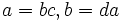$a = bc, b = da$. By the definition of multiplicatively monotone, we get: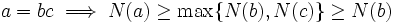$a = bc \implies N(a) \ge \max \{ N(b), N(c) \} \ge N(b)$.

Similarly: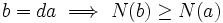$b = da \implies N(b) \ge N(a)$.